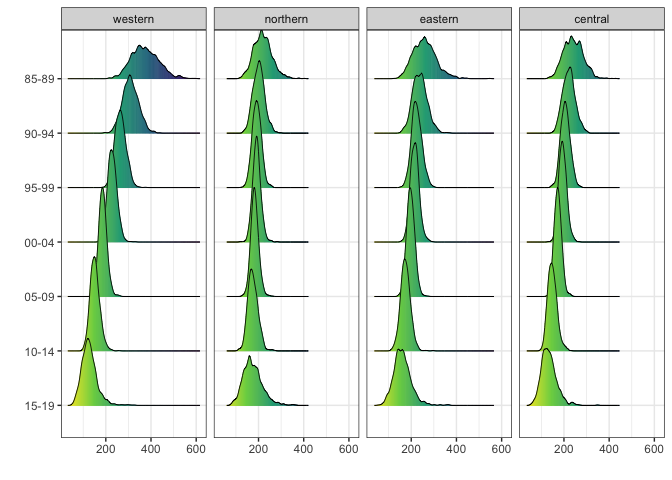# A Case Study of Estimating Subnational U5MR using Cluster-level Methods

#### 2021/01/31

In this vignette, we will discuss the use of cluster-level models using the SUMMER package. We will use the simulated surveys in the DemoData dataset in the package. The DemoData object is a list that contains full birth history data from simulated surveys with stratified cluster sampling design, similar to most of the DHS surveys. It has been pre-processed into the person-month format, where for each list entry, each row represents one person-month record. Each record contains columns for the cluster ID (clustid), household ID (id), strata membership (strata) and survey weights (weights). The region and time period associated with each person-month record has also been computed and saved in the dataset. Raw DHS data can be processed into this format using the getBirths function. More details of the data processing step and person-month format are discussed in the package vignette A Case Study of Estimating Subnational U5MR using Smoothed Direct Methods.

We first load the package and data. We will use the dplyr package in some data processing steps.

library(SUMMER)
data(DemoData)
library(dplyr)

We now describe the fitting of the cluster level model for U5MR. For this simulated dataset, there is no stratification within regions, so we treat the region variable as strata. To fit the cluster-level model, we calculate the number of person-months and number of deaths for each cluster, time period, and age group. We first create the data frame using the getCounts function for each survey and combine them into a single data frame.

The model fitting function smoothCluster expects columns with specific names. We rename the cluster ID and time period columns to be ‘cluster’ and ‘years’. The response variable is ‘Y’ and the binomial total is ‘total’.

counts.all <- NULL
for (i in 1:length(DemoData)) {
vars <- c("clustid", "region", "time", "age")
counts <- getCounts(DemoData[[i]][, c(vars, "died")], variables = "died", by = vars,
drop = TRUE)
counts <- counts %>% mutate(cluster = clustid, years = time, Y = died)
counts$survey <- names(DemoData)[i] counts.all <- rbind(counts.all, counts) } With the created data frame, we fit the cluster-level model using the smoothCluster function. Notice that here we need to specify the age groups (age.groups), the length of each age group (age.n) in months, and how the age groups are mapped to the temporal random effects (age.rw.group). In the default case, age.rw.group = c(1, 2, 3, 3, 3, 3) means the first two age groups each has its own temporal trend, the the following four age groups share the same temporal trend. We start with the default temporal model of random walk or order 2 on the 5-year periods in this dataset (with real data, we can use a finer temporal resolution). We add survey iid effects to the model as well. periods <- c("85-89", "90-94", "95-99", "00-04", "05-09", "10-14") fit.bb <- smoothCluster(data = counts.all, Amat = DemoMap$Amat,
family = "betabinomial",
year_label = c(periods, "15-19"),
age.groups = c("0", "1-11", "12-23", "24-35", "36-47", "48-59"),
age.n = c(1,11,12,12,12,12),
age.rw.group = c(1,2,3,3,3,3),
time.model = "rw2", survey.effect = TRUE)
## This is INLA_20.03.17 built 2020-12-08 17:33:40 UTC.
## See www.r-inla.org/contact-us for how to get help.
## To enable PARDISO sparse library; see inla.pardiso()
## ----------------------------------
## Cluster-level model
##   Main temporal model:        rw2
##   Spatial effect:             bym2
##   Interaction temporal model: rw2
##   Interaction type:           4
##
##   Number of age groups: 6
##   Number of age-specific trends per stratum: 3
##   Stratification: no, strata variable not in the input
##   Survey effect: yes
## ----------------------------------

In this example, we do not have urban/rural strata in the dataset. When further within area stratification exists, we may also choose to model stratum-age specific temporal trends using the strata.time.effect argument. We also do not have any bias adjustment in this simple model. If the ratio adjustments to U5MR are known, they could be entered with the bias.adj and bias.adj.by arguments when fitting the model.

Posterior samples from the model are taken and summarized using the getSmoothed function. For models with a large number of areas and time points, this step may take some time to compute. The save.draws argument makes it possible to save the raw posterior draws, so that we can use them again in other functions or recompute different posterior credible intervals.

est.bb <- getSmoothed(fit.bb, nsim = 1000, CI = 0.95, save.draws = TRUE)
summary(est.bb)
##                   Length Class      Mode
## overall             13   SUMMERproj list
## stratified          12   SUMMERproj list
## draws             1000   -none-     list
## draws.est           28   -none-     list
## draws.est.overall   28   -none-     list

For example, to recompute the posterior CI directly using the existing draws:

est.bb.90CI <- getSmoothed(fit.bb, nsim = 1000, CI = 0.95, draws = est.bb$draws) ## Model comparisons There are many options to change the model components. For example, we can use a different temporal component in the space-time interaction model. The default interaction model assumes the temporal component to follow the same model as the main temporal trend (so in the example above, random walk or order 2) and interact with a spatial ICAR process. Here we specify a different model where the space-time interaction is assumed to follow an AR(1) interacting with an ICAR process (via the st.time.model argument). We also add region-specific random slopes in time (via pc.st.slope.u and pc.st.slope.alpha; these two parameters specify the PC priors for the random slopes). fit.bb.ar1 <- smoothCluster(data = counts.all, Amat = DemoMap$Amat,
family = "betabinomial",
year_label = c(periods, "15-19"),
age.groups = c("0", "1-11", "12-23", "24-35", "36-47", "48-59"),
age.n = c(1,11,12,12,12,12),
age.rw.group = c(1,2,3,3,3,3),
time.model = "rw2", survey.effect = TRUE,
st.time.model = "ar1", type.st = 4,
pc.st.slope.u = 1, pc.st.slope.alpha = 0.1)
## ----------------------------------
## Cluster-level model
##   Main temporal model:        rw2
##   Spatial effect:             bym2
##   Interaction temporal model: ar1
##   Interaction type:           4
##   Interaction random slopes:  yes
##   Number of age groups: 6
##   Number of age-specific trends per stratum: 3
##   Stratification: no, strata variable not in the input
##   Survey effect: yes
## ----------------------------------
est.bb.ar1 <- getSmoothed(fit.bb.ar1, nsim = 1000, CI = 0.95)
## Starting posterior sampling...
## Cleaning up results...
## No stratification in the model. Set all weights to 1.

Since we fit a non-stratified model, the stratified object and overall object in the returned list are the same. We can plot the estimates (or a subset of the estimates) using the S3 class plot function. For example, we plot the estimates for the first model with RW(2) interactions.

library(ggplot2)
plot(est.bb$overall, per1000 = TRUE, plot.CI = TRUE) + facet_wrap(~region, ncol = 4) + ylim(0, 600) + theme(axis.text.x = element_text(angle = 45, hjust = 1))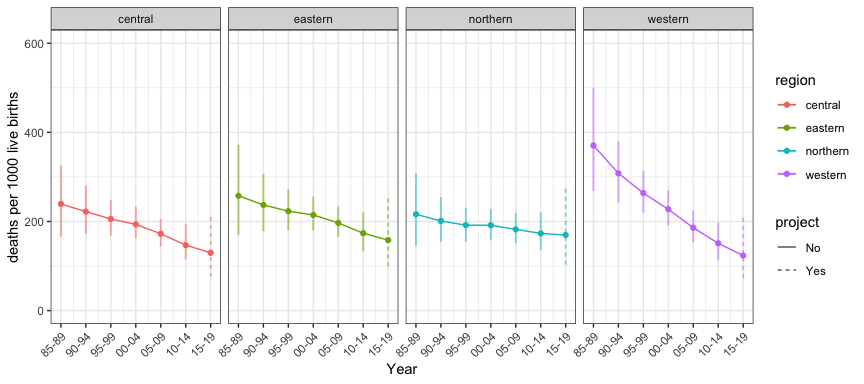In comparison, we plot the estimate from the AR(1) interaction model on the same scale. It can be seen that it induces stronger shrinkage for the region-specific slopes towards the shared trend. plot(est.bb.ar1$overall, per1000 = TRUE, plot.CI = TRUE) + facet_wrap(~region, ncol = 4) +
ylim(0, 600) + theme(axis.text.x = element_text(angle = 45, hjust = 1))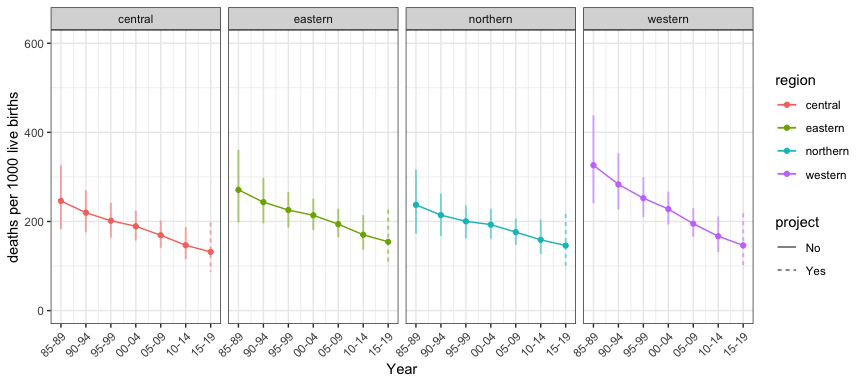## More visualizations

We show the estimates on the map using the mapPlot function. We use the DemoMap from the package containing geographic data from the 1995 Uganda Admin 1 regions (but be aware the data are simulated and not correspond to Uganda child mortality).

data(DemoMap)
mapPlot(est.bb$overall, geo = DemoMap$geo, by.data = "region", by.geo = "REGNAME",
is.long = TRUE, variables = "years", values = "median",
ncol = 4, direction = -1, per1000 = TRUE, legend.label = "U5MR")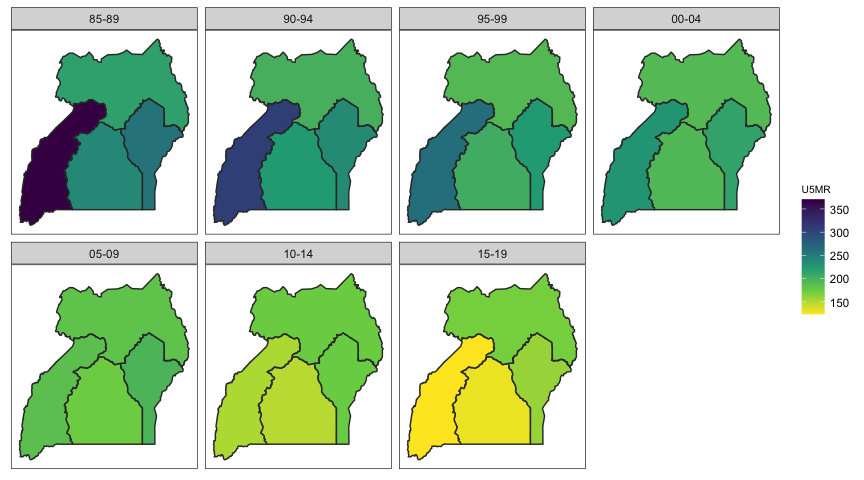We can add hatching to indicate uncertainty of the estimates using hatchPlot with similar syntax.

hatchPlot(est.bb$overall, geo = DemoMap$geo, by.data = "region", by.geo = "REGNAME",
is.long = TRUE, variables = "years", values = "median",
lower = "lower", upper = "upper", hatch = "red",
ncol = 4, direction = -1, per1000 = TRUE, legend.label = "U5MR")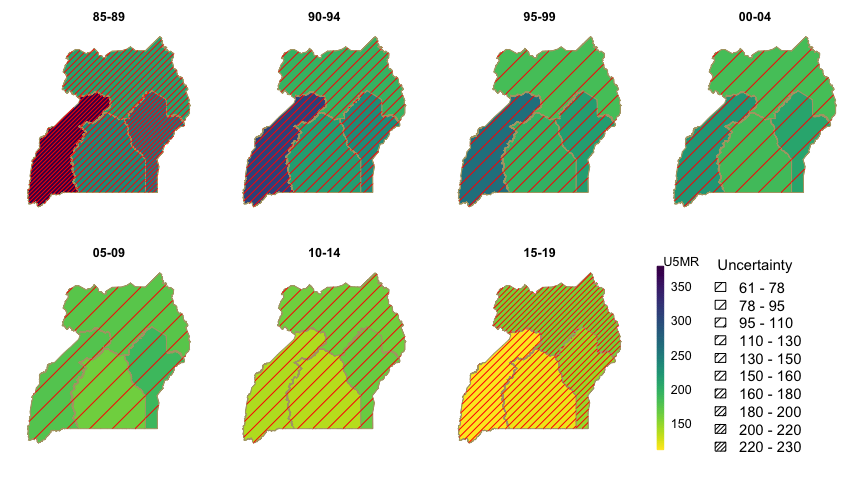Next we show the posterior densities of the estimates, where each each panel correspond to one time period, and the regions are ordered by the posterior median of estimates in the last year (specified by the order = -1 argument).

dens <- ridgePlot(draws = est.bb, Amat = DemoMap$Amat, year_plot = c(periods, "15-19"), ncol = 4, per1000 = TRUE, order = -1, direction = -1) dens$g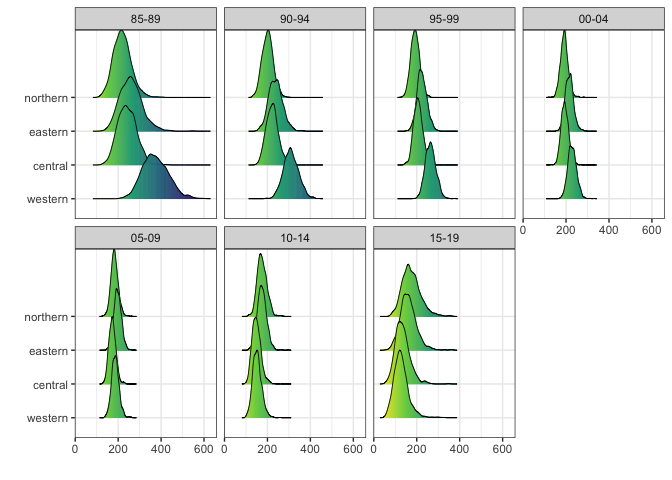It can also be plotted for each region over time as well.

dens <- ridgePlot(draws = est.bb, Amat = DemoMap$Amat, year_plot = c(periods, "15-19"), ncol = 4, per1000 = TRUE, by.year = FALSE, direction = -1) dens$g